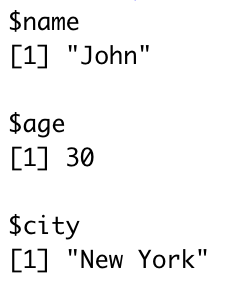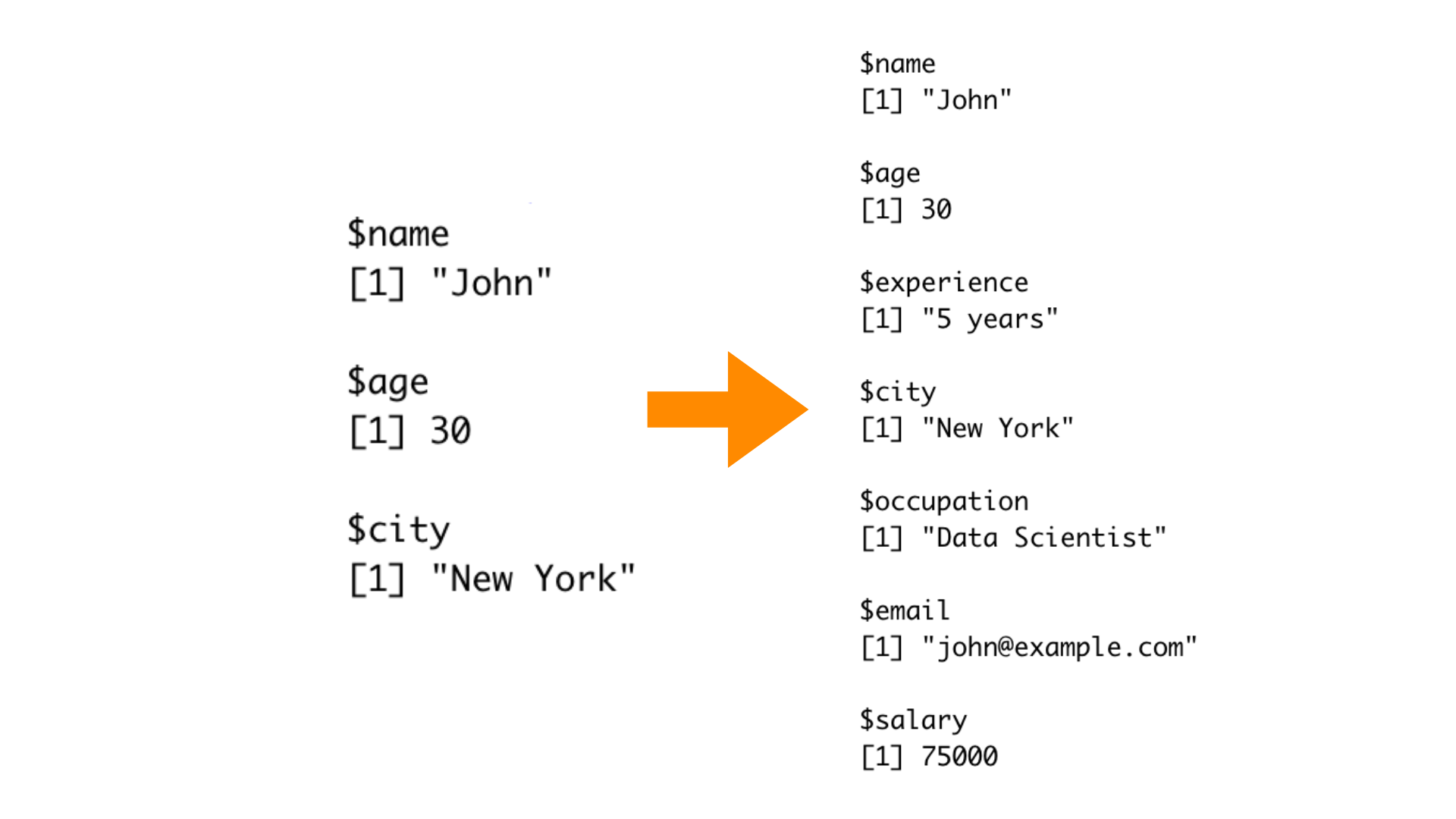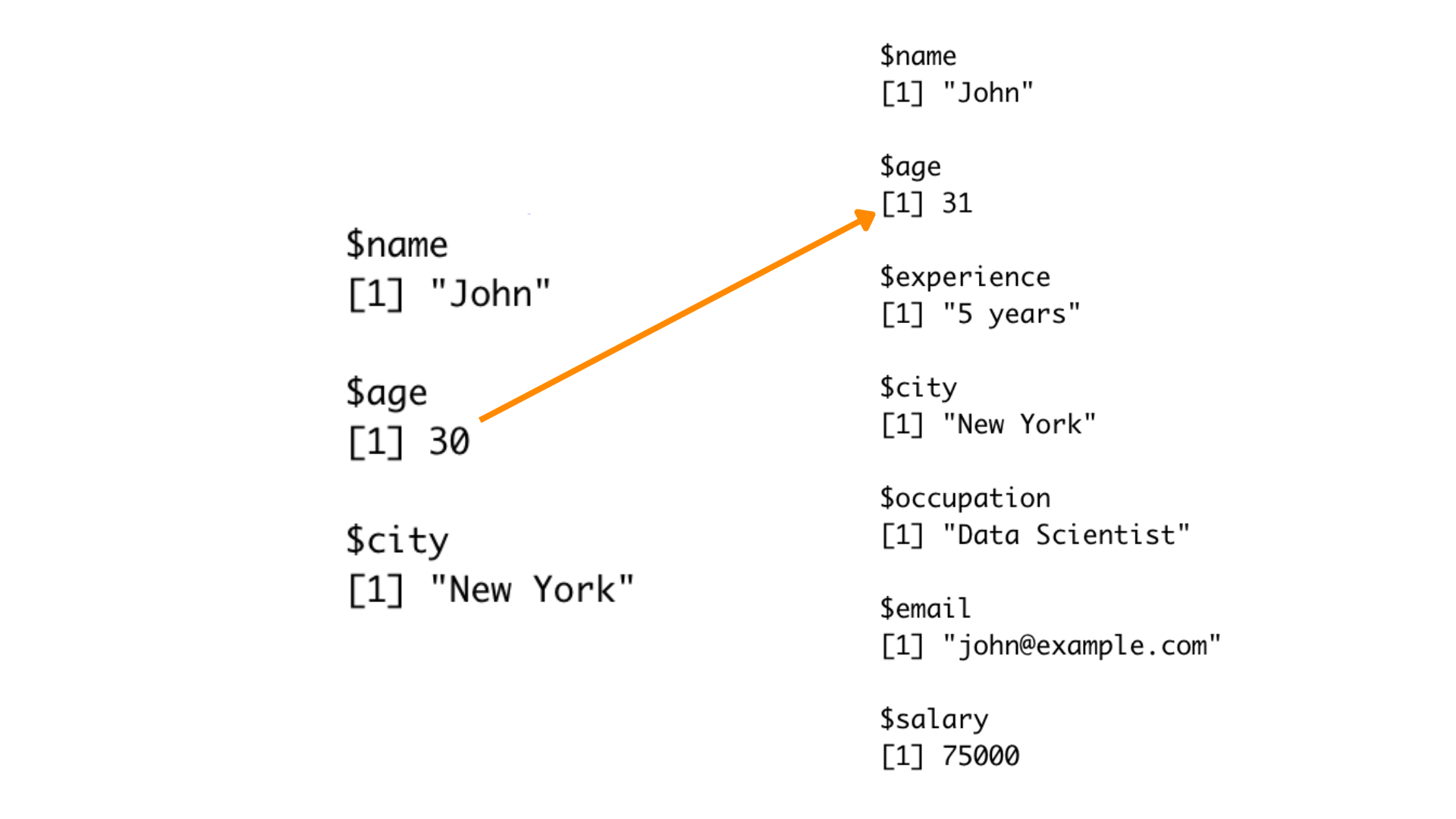# How to Append to List in R? | 5 Methods (With Examples)

Lists are one of the fundamental data structures in R, allowing you to store heterogeneous data types and organize them in a flexible manner. In this article, we will explore how to add or append elements to lists in R, an essential skill for any R programmer.

## Understanding Lists in R

Before we jump into adding or appending elements, let's take a moment to understand what lists are in R.

A list is an ordered collection of objects or data of different types, such as vectors, data frames, or even other lists. This ability to store different data types within a single list makes it a versatile container for complex data structures.

To create a list in R, you can use the `list()` function. Here's a basic example:

```my_list <- list(name = "John", age = 30, city = "New York")
```Here, we've generated a list named `my_list` that contains three elements: a character vector called name, a numeric value called age, and another character vector called city. Let's now look at how to add or append elements to this list.

## Adding Elements to a List

Adding elements to a list in R can be accomplished using various techniques, depending on your specific needs. Here are five different methods to add elements to an existing list:

### 1. Using the \$ operator

You can use the \$ operator to add new elements to a list or modify existing ones. Simply specify the name of the new element and assign a value to it. Here's an example:

```my_list\$occupation <- "Data Scientist"
```

Now, our my_list contains an additional element called "occupation."

### 2. Using the c() function

To append multiple elements to a list simultaneously, you can use the c() function, which concatenates vectors. First, create a new list containing the elements you want to add, and then use c() to combine the original list and the new list. Here's how it works:

```new_elements <- list(email = "[email protected]", salary = 75000)

my_list <- c(my_list, new_elements)
```

Now, `my_list` includes two new elements: "email" and "salary."

### 3. Using the append() function

We can use the append() function to add or append elements into a list in R. It takes three arguments: the original list, the elements to be added, and the position at which to add them. Here's an example:

```my_list <- append(my_list, list(experience = "5 years"), after = 2)
```In this example, the "experience" element is added after the second position in the list.

### 4. Using the `rlist` library

The rlist library provides convenient functions for working with lists, making it easier to manipulate list structures.

Here's an example of how to use rlist to add elements to a list:

```library(rlist)

new_elements <- list(phone = 8856732415)

my_list <- list.append(my_list, new_elements)
```

In this example, we use `list.append()` from the rlist library to add the elements from `new_elements` to `my_list`.

Using the rlist library simplifies the process of adding elements to a list and can be particularly helpful when you need to work with complex lists and perform various list operations.

To add multiple elements to a list in R, we can use the list() function and the c() function together.

You can create a new list containing the elements you want to add and then use the c() function to combine the original list and the new list. Here's an example:

```new_elements <- list(hobby = "Reading", skills = c("R programming", "Data analysis"))

my_list <- c(my_list, new_elements)
```

In this example, the new_elements list contains two elements, "hobby" and "skills," each with their respective values. These elements are then added to the my_list, expanding its content.

### Modifying Existing Elements

In addition to adding new elements, you can also modify existing elements within a list. To do this, simply access the element using the \$ operator or by its index and assign a new value to it. Here's an example:

```my_list\$age <- 31
```Here, we've updated the "age" element to 31.

## Conclusion

Lists are a fundamental data structure in R, providing a flexible way to store and manipulate heterogeneous data. Understanding how to add or append elements to lists is crucial for any R programmer, as it allows you to dynamically build and update complex data structures. Whether you use the \$ operator, the c() function, or the append() function, you now have the tools to efficiently manage your lists in R.

### FavTutor - 24x7 Live Coding Help from Expert Tutors!